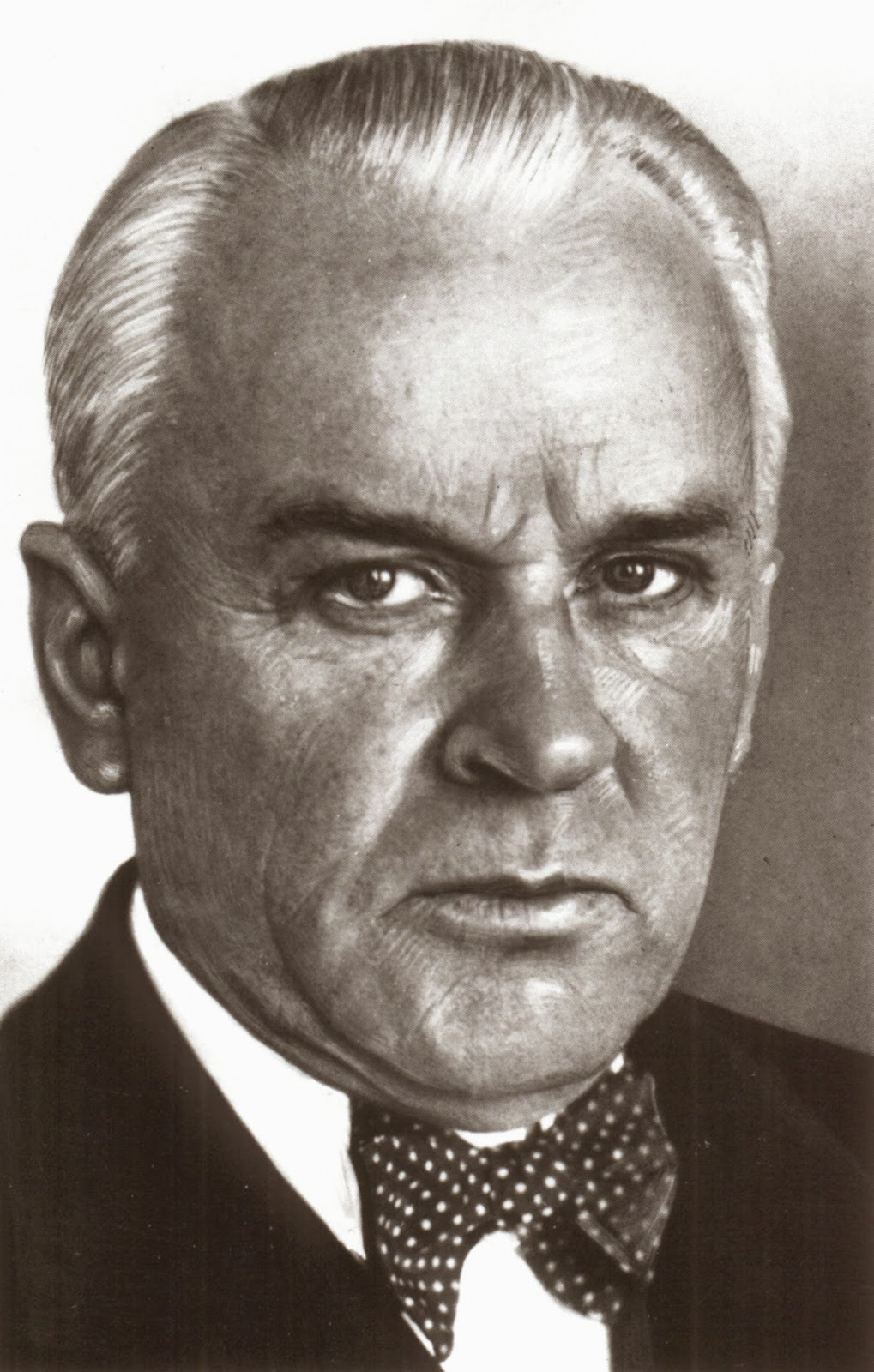## söndag 30 mars 2014

### Photoelectricity: Millikan vs EinsteinThe American physicist Robert Millikan received the Nobel Prize in 1923 for (i) experimental determination of the charge $e$ of an electron and (ii) experimental verification of Einstein's law of photoelectricity awarded the 1921 Prize.

Millikan started out his experiments on photoelectricity with the objective of disproving Einstein's law and in particular the underlying idea of light quanta. To his disappointment Millikan found that according to his experiments Einstein's law in fact was valid, but he resisted by questioning the conception of light-quanta even in his Nobel lecture:
• In view of all these methods and experiments the general validity of Einstein’s equation is, I think, now universally conceded, and to that extent the reality of Einstein’s light-quanta may be considered as experimentally established.
• But the conception of localized light-quanta out of which Einstein got his equation must still be regarded as far from being established.
• Whether the mechanism of interaction between ether waves and electrons has its seat in the unknown conditions and laws existing within the atom, or is to be looked for primarily in the essentially corpuscular Thomson-Planck-Einstein conception as to the nature of radiant energy is the all-absorbing uncertainty upon the frontiers of modern Physics.
Millikan's experiments consisted in subjecting a metallic surface to light of different frequencies $\nu$ and measuring the resulting photovoltic current determining a smallest frequency $\nu_0$ producing a current and (negative) stopping potential required to bring the current to zero for frequencies $\nu >\nu_0$. Millikan thus measured $\nu_0$ and $V$ for different frequencies $\nu > \nu_0$ and found a linear relationship between $\nu -\nu_0$ and $V$, which he expressed as
• $\frac{h}{e}(\nu -\nu_0)= V$,
in terms of the charge $e$ of an electron which he had already determined experimentally, and the constant $h$ which he determined to have the value $6.57\times 10^{-34}$. The observed linear relation between $\nu -\nu_0$ and $V$ could then be expressed as
• $h\nu = h\nu_0 +eV$
which Millikan had to admit was nothing but Einstein's law with $h$ representing Planck's constant.

But Millikan could argue that, after all, the only thing he had done was to establish a macroscopic linear relationship between $\nu -\nu_0$ and $V$, which in itself did not give undeniable evidence of the existence of microscopic light-quanta. What Millikan did was to measure the current for different potentials of the plus pole receiving the emitted electrons under different exposure to light and thereby discovered a linear relationship between frequency $\nu -\nu_0$ and stopping potential $V$ independent of the intensity of the light and properties of the metallic surface.

By focussing on frequency and stopping potential Millikan could make his experiment independent of the intensity of incoming light and of the metallic surface, and thus capture a conversion between light energy and electron energy of general significance.

But why then should stopping potential $V$ scale with frequency $\nu - \nu_0$, or $eV$ scale with frequency $h(\nu - \nu_0)$? Based on the analysis on Computational Blackbody Radiation the answer would be that $h\nu$ represents a threshold energy for emission of radiation in Planck's radiation law and $eV$ represents a threshold energy for emission of electrons, none of which would demand light quanta.